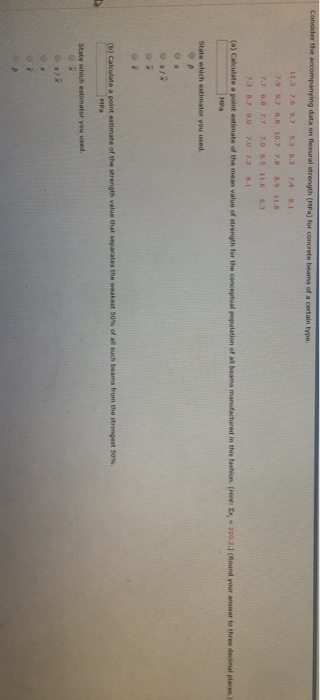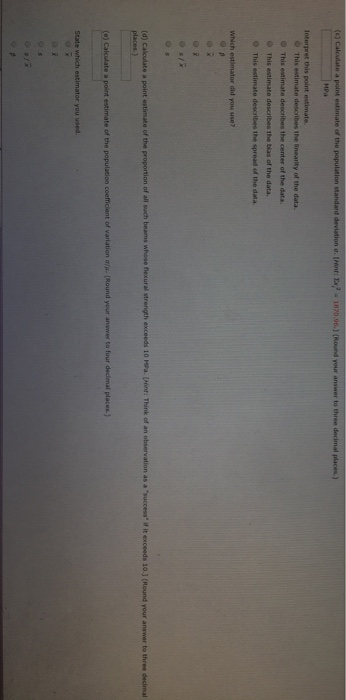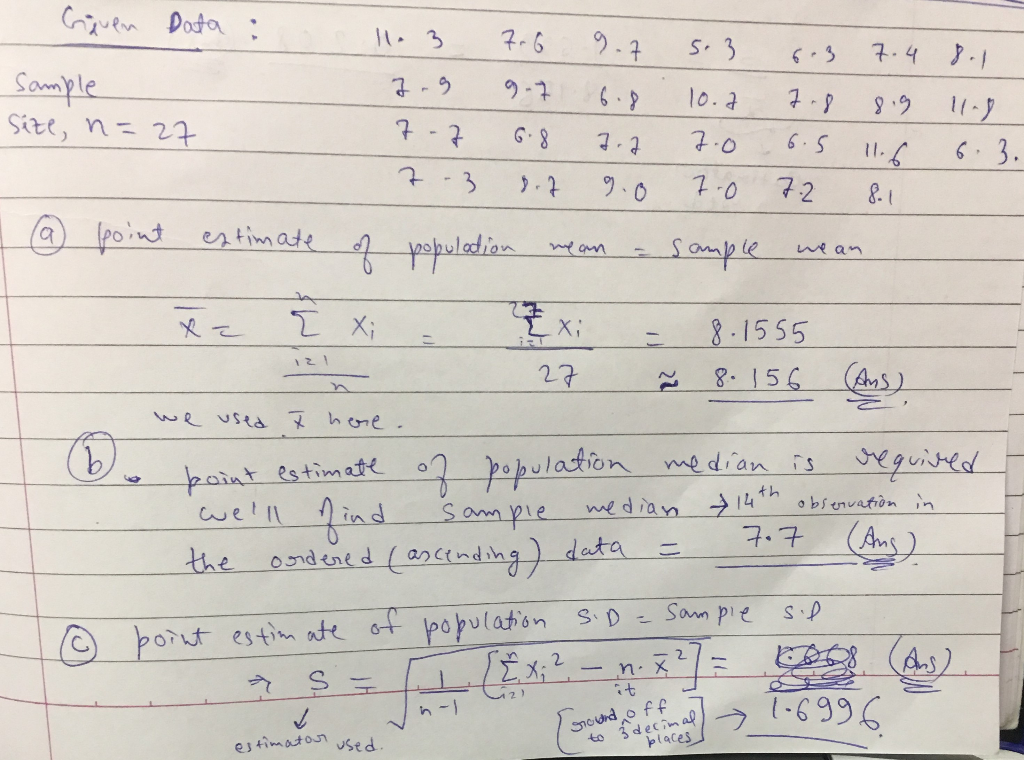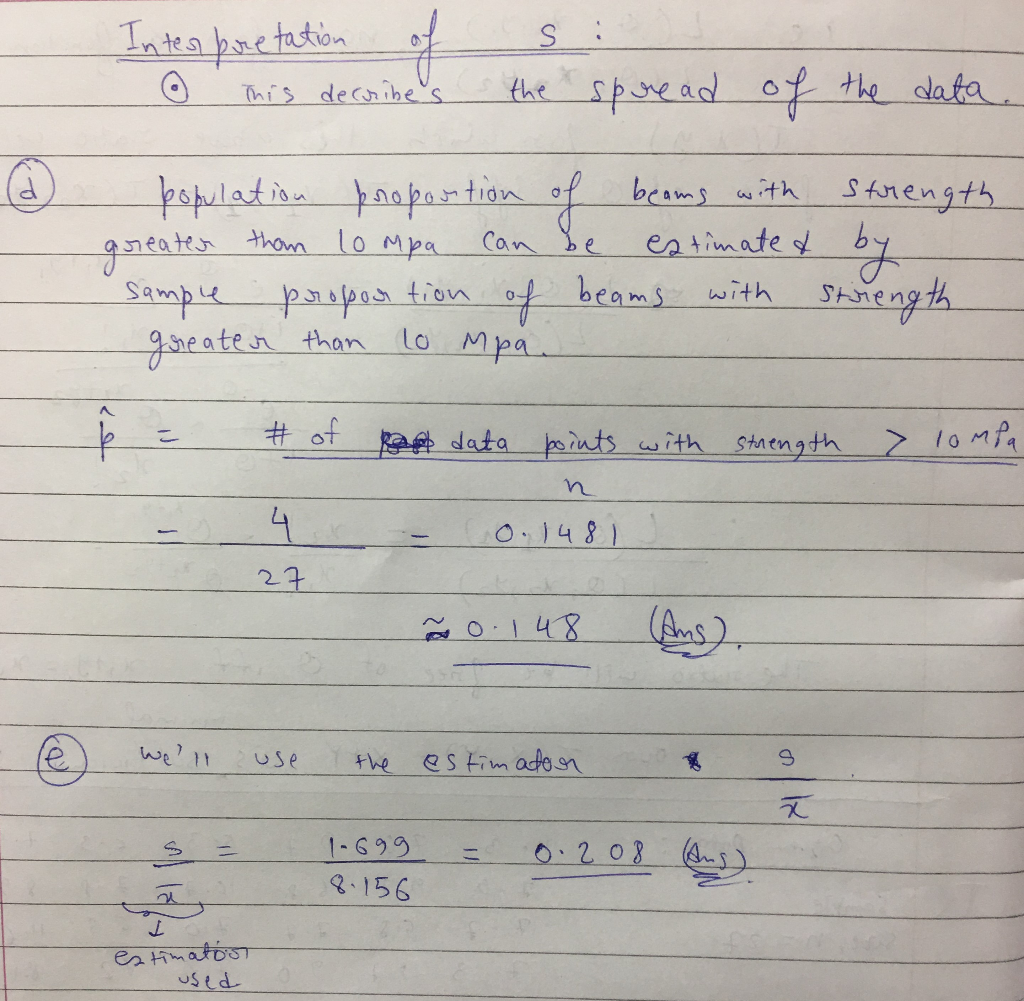# Hello The wuestion is in the two pictures in the bottom, thank u! We were unable...

Hello The wuestion is in the two pictures in the bottom, thank u!We were unable to transcribe this image
e of the Interpret this point estimate This estimate describes the linearity of the data. This estimate, describes the center of the data. This estimate describes the bias of the data. This estimate describes the spread o the data. Which estimator did you use? (d) Calculate a point estimate of the proportion of all such beams whose flexural strength exceeds 10 MPa. (Hint: Think of an observation as a "success" if it exceeds 10.3 (Round your answer to three decimal

following two images contain the answers and all the explanation required.So check them out!!

image 1:image 2:thats all.Do thumbs up if this helped

cheers.

#### Earn Coin

Coins can be redeemed for fabulous gifts.